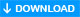# fputcsv(): Writing data to file

We can prepare data to store in a comma separated value ( CSV ) file by using fputcsv() function. Here is the syntax
``fputcsv(filepointer, array(fields), delimiter, enclosure)``
Last two parameters are option.

filepointer: previously opened file pointer

array(fields) : The data array

delimiter : by default it is comma ( , ) , you can change to a new one

enclosure : One character can be used to enclose data

## From array to CSV file

We will use one multidimensional array and then use the same data to store in a csv file by using fputcsv function.
``````<?php
\$a= array(array("path"=>"php_tutorial","section"=>"php"),
array("path"=>"sql_tutorial","section"=>"sql"),
array("path"=>"javascript_tutorial","section"=>"js"),
array("path"=>"html_tutorial","section"=>"html"),
);

\$f_pointer=fopen("website.csv","w"); // file pointer

foreach (\$a as \$fields) {
fputcsv(\$f_pointer, \$fields);
}
fclose(\$f_pointer);
?>
``````

## From database to CSV file

Let us read the records of our student table ( download the sql_dump of student table here ) and create a csv file using the data.
``````<?php
require "config.php"; // database connection
\$fp = fopen('student.csv', 'w');

\$query = \$dbo->prepare("select *  FROM student");
\$query->execute();

for(\$i=0; \$row = \$query->fetch(PDO::FETCH_NUM); \$i++){
fputcsv(\$fp, \$row);
}

fclose(\$fp);  // Closing file pointer
?>
``````

We will modify file header and post the csv file to the browser so user can download it and save in the local computer.
``````<?Php
require "config.php"; // Database Connetion
\$sql= "SELECT * from student ";

if(strlen(\$sql) <6){echo "No Query ";
exit;
}

\$filename='student.csv';
\$fp = fopen('php://output', 'w');

\$STH = \$dbo->prepare(\$sql);
\$STH->execute();

\$first_row = \$STH->fetch(PDO::FETCH_ASSOC);
fputcsv(\$fp, array_values(\$first_row)); // put the first row

while (\$row = \$STH->fetch(PDO::FETCH_NUM))  {
fputcsv(\$fp,\$row); // push the rest
}
fclose(\$fp);
?>``````
In above code config.php file stores the database connection and login details.

Using MySQLI database connectin
``````<?Php
require "config-mysqli.php"; // Database Connetion

\$sql= "SELECT * FROM  student ";  // Query

\$filename='student.csv';  // file name
\$fp = fopen('php://output', 'w');

if(\$stmt = \$connection->query("\$sql")){
\$no_of_columns=\$stmt->field_count;
\$row = mysqli_fetch_assoc(\$stmt);
fputcsv(\$fp, array_values(\$row)); // put the first row
while (\$row = \$stmt->fetch_array(MYSQLI_NUM)) {
fputcsv(\$fp, \$row);
}
fclose(\$fp);
}else{
echo \$connection->error;
}
?>``````

## Storing visitor data in a CSV file.

We can store visitors data like ip address, referrer, browser details and time of visit in a csv file.

Read how the visitors details are stored in a CSV file by using fputcsv function

Note the way we are opening the file. When we are storing the visitor details we have to add one line each time a new visitor details are to be stored. When we are storing the student records we are opening the file and storing all data in one go and then closing it.student.csvSubscribe to our YouTube Channel here

## Subscribe

* indicates required
Subscribe to plus2netplus2net.com

PHP String Functions

Post your comments , suggestion , error , requirements etc here .